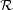### A GLOSSARY

 Variable Definition α Spectral index β Luminosity power law index γ Source counts index λ Observing wavelength (m) ν Observing frequency (MHz) Δ ν Observing bandwidth (MHz) Δ νScint Scintillation bandwidth (MHz) σs Root mean square fluctuations in the time series τs Scattering timescale (s) Θ Position angle (∘) Ωm Energy density of matter ΩΛ Energy density of dark energy B(ℓ)|| Magnetic field parallel to the line of sight (G) D Telescope diameter (m) DM Dispersion measure (cm−3 pc) DMe Dispersion measure excess (cm−3 pc) DMIGM Dispersion measure from the intergalactic medium (cm−3 pc) DMMW Dispersion measure from the Milky Way (cm−3 pc) dL Luminosity distance (Gpc)Fluence (Jy ms) G Antenna gain (K Jy−1) L Luminosity (W) NDM Number of DM trials Nt Number of time trials Nν Number of frequency channels ne Electron number density (cm−3)FRB event rate (FRBs sky−1 day−1) RM Rotation measure (rad m−2) SM Scattering measure (kpc m−20/3) S/N Signal-to-noise ratio Speak Peak flux density (Jy) TB Brightness temperature (K) Tsys System temperature (K) Δ t Dispersive delay (s) Δ tDM Dispersive delay across an individual frequency channel (s) Δ tDMerr Dispersive delay due to dedispersion at a slightly incorrect DM (s) tsamp Sampling time (s) x(z) Ionization fraction as a function of redshift W Pulse width (s) Weq Equivalent width (s) Wint Intrinsic pulse width (s)